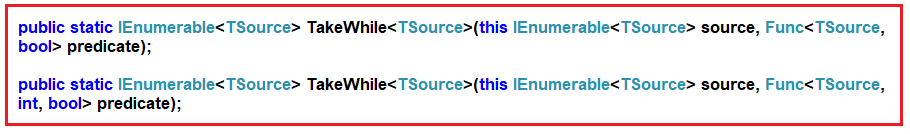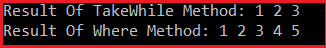# LINQ TakeWhile Method in C#

## LINQ TakeWhile Method in C# with Examples

In this article, I will discuss the LINQ TakeWhile Method in C# with Examples. Please read our previous article discussing the LINQ Take Method in C# with Examples. As part of this article, we will discuss the following pointers related to the LINQ TakeWhile Method in C#.

1. What is the LINQ TakeWhile Method?
2. Examples to Understand TakeWhile Method using both Method and Query Syntax.
3. Understanding TakeWhile Method with Filtering Method.
4. What is the difference between the Where and TakeWhile Methods in LINQ?
5. When to Use the TakeWhile Method in C#?
##### What are the needs and uses of the LINQ TakeWhile Method in C#?

The LINQ TakeWhile Method in C# fetches all the elements from a data source, a sequence, or a collection until a specified condition is true. Once the condition fails, the TakeWhile method will not check the rest of the elements in the data source, even though the condition is true for some of the remaining elements.

The LINQ TakeWhile Method belongs to System.Linq namespace can be applied to any type implementing IEnumerable<T>. TakeWhile is especially useful in scenarios where you want to extract a contiguous portion from the start of a sequence based on some condition. You need to remember that, like the Take Method, the LINQ TakeWhile Method will not change the positions of the elements in the data source.

TakeWhile takes a predicate function that specifies the condition to test each element. It includes elements in the result as long as the condition is true. Once an element is encountered for which the condition is false, TakeWhile stops including elements. There are two overloaded versions available for this TakeWhile Method in C#. They are as shown in the below image.The First version of the LINQ TakeWhile method returns elements from a sequence as long as the specified condition is true. Once the condition fails, it will not check the remaining elements in the data source.

If the given condition is true, the Second Overloaded version of the TakeWhile method returns elements from a sequence. The second parameter of the function represents the index of the source elements. The element’s index can be used in the logic of the predicate function.

##### Example to Understand LINQ TakeWhile Method in C#:

Let us see an example of understanding the LINQ TakeWhile Method in C# using Method syntax and Query Syntax. In the following example, we created a data source that contains numbers from 1 to 10. Then, we fetch the records from the data source using the TakeWhile method with the condition that the number is less than 6. You need to remember that no such operator called take while is available in LINQ to write the query syntax. So, here, we need to use the mixed syntax shown in the example below.

```using System;
using System.Collections.Generic;
using System.Linq;

namespace LINQDemo
{
class Program
{
static void Main(string[] args)
{
//Data Source
List<int> numbers = new List<int>() { 1, 2, 3, 4, 5, 6, 7, 8, 9, 10 };

//Fetch Numbers which are less than 6 using TakeWhile Method
//Using Method Syntax
List<int> ResultMS = numbers.TakeWhile(num => num < 6).ToList();

//Using Query Syntax
List<int> ResultQS = (from num in numbers
select num).TakeWhile(num => num < 6).ToList();

//Accessing the Result using Foreach Loop
foreach (var num in ResultMS)
{
Console.Write(\$"{num} ");
}

}
}
}```

Output: 1 2 3 4 5

##### Key Points
• Use Case: TakeWhile is used when extracting a sequence of elements that meet a certain condition from the start of a collection.
• Deferred Execution: Like many LINQ methods, TakeWhile uses deferred execution.
• Order-Dependent: The behavior of TakeWhile is dependent on the order of the elements in the sequence. It includes elements until it finds one that does not satisfy the condition.
• Difference from Where: Unlike Where, which filters out elements that don’t meet the condition throughout the entire sequence, TakeWhile only includes elements until an element fails the condition and then stops evaluating further.
##### LINQ TakeWhile Method along with the Where Filtering Method in C#.

Now, you may have one question in your mind: the same thing we can achieve using the LINQ Where extension method. For a better understanding, please look at the following example, which uses the LINQ Where method.

```using System;
using System.Collections.Generic;
using System.Linq;

namespace LINQDemo
{
class Program
{
static void Main(string[] args)
{
//Data Source
List<int> numbers = new List<int>() { 1, 2, 3, 4, 5, 6, 7, 8, 9, 10 };

//Fetch Numbers which are less than 6 using Where Method
//Using Method Syntax
List<int> ResultMS = numbers.Where(num => num < 6).ToList();

//Using Query Syntax
List<int> ResultQS = (from num in numbers
where num < 6
select num).ToList();

//Accessing the Result using Foreach Loop
foreach (var num in ResultQS)
{
Console.Write(\$"{num} ");
}

}
}
}
```

Output: 1 2 3 4 5

##### What is the Difference Between the Where and TakeWhile Method in LINQ?

Before understanding the difference between the LINQ Where and TakeWhile Extension Methods, let us first modify the Main method of the Program class as shown below and look at the output; we move the values 4 and 5 to the end of the collection or in the data source. That means the data source now stores the records in random order.

```using System;
using System.Collections.Generic;
using System.Linq;

namespace LINQDemo
{
class Program
{
static void Main(string[] args)
{
//Data Source: Numbers are Stored in Randon Order
List<int> numbers = new List<int>() { 1, 2, 3, 6, 7, 8, 9, 10, 4, 5 };

//Using TakeWhile Method to Fetch Numbers which are less than 6
List<int> Result1 = numbers.TakeWhile(num => num < 6).ToList();
Console.Write("Result Of TakeWhile Method: ");
foreach (var num in Result1)
{
Console.Write(\$"{ num} ");
}
Console.WriteLine();

//Using Where Method to Fetch Numbers which are less than 6
List<int> Result2 = numbers.Where(num => num < 6).ToList();
Console.Write("Result Of Where Method: ");
foreach (var num in Result2)
{
Console.Write(\$"{ num} ");
}

}
}
}
```
###### Output:As you can see in the above output, the LINQ TakeWhile method fetches the values 1, 2, and 3, while the LINQ Where method fetches the values 1, 2, 3, 4, and 5.

So, the difference between them is that the TakeWhile Extension Method checks the conditions from the beginning of the data source. As long as the condition is true, it fetches the data. So, in our example, the condition is true for the first three elements. So, the TakeWhile method fetches the first three elements. When it checks the fourth element, the condition becomes false. So, from that point, it will not check the rest of the elements in the data source even though some of the elements (i.e., 4 and 5 are present at the end of the collections) satisfy the condition.

On the other hand, the LINQ Where Extension Method checks each and every element present in the collection. The elements which satisfy the given condition will be returned as part of the result. It does not matter the position of the elements in the sequence.

##### Example to Understand TakeWhile Method with String Collection:

In the following example, we have a collection of names. Here, we need to return the names from the beginning until a name is hit that does not have a length greater than 3 characters. We should and must go with the TakeWhile Method instead of the Where Extension method for this requirement.

```using System;
using System.Collections.Generic;
using System.Linq;

namespace LINQDemo
{
class Program
{
static void Main(string[] args)
{
List<string> names = new List<string>() { "Sara", "Rahul", "John", "Pam", "Priyanka" };

List<string> namesResult = names.TakeWhile(name => name.Length > 3).ToList();

foreach (var name in namesResult)
{
Console.Write(\$"{ name} ");
}

}
}
}```

Output: Sara Rahul John

##### Example to Understand TakeWhile Method, which takes Index as a Parameter:

Let us use the other overloaded version of the LINQ TakeWhile Method, which takes an index as a parameter. Here, in the following example, we check the length of the name should be greater than its index position. So, the first element index is 0, the second element index is 1, the third element index is 2, and so on. Then, we check whether the length of the value is greater than its index position. If it exceeds its index position, it will return true. Else, it will return false. In the data source, the fourth element, i.e., Pam, has a length of 3, and its index position is 3. So, here, the condition is false; hence, it will not check the rest of the elements in the data source. As a result, it only fetches the first three elements from the sequence.

```using System;
using System.Collections.Generic;
using System.Linq;

namespace LINQDemo
{
class Program
{
static void Main(string[] args)
{
List<string> names = new List<string>() { "Sara", "Rahul", "John", "Pam", "Priyanka" };

List<string> namesResult = names.TakeWhile((name, index) => name.Length > index).ToList();

foreach (var name in namesResult)
{
Console.Write(\$"{ name} ");
}

}
}
}
```

Output: Sara Rahul John

##### When to use the LINQ TakeWhile Method in C#?

The LINQ TakeWhile method in C# is particularly useful in scenarios where you need to process or extract a contiguous subset from the start of a sequence based on a specified condition. Here are some typical use cases:

• Extracting Initial Sequence Elements: When you want to take elements from the beginning of a collection until a certain condition is no longer true. For instance, reading lines from a log file until you reach a line that indicates an error or a specific event.
• Sequential Data Processing: In ordered datasets, you need to process elements in sequence until a condition fails. For example, processing time-series data or sequential readings until a value falls below a threshold.
• Data Analysis: In data analysis tasks, TakeWhile can be used to analyze parts of a dataset until a certain criterion is met, like analyzing sales data up to a certain date or threshold.
• Stream Processing: When dealing with data streams (like from a sensor or a live feed), TakeWhile can process data continuously until a specific condition is met.
• Conditional Parsing: In parsing tasks, you need to parse through a document or dataset and extract information up until a certain marker or condition is encountered.
• Handling Ordered Collections: TakeWhile is especially suitable for collections where the order of elements is meaningful and you are interested in a subset based on a condition from the beginning.
• Early Termination in Algorithms: When implementing algorithms that can terminate early based on a condition, TakeWhile can be used to simplify the logic by taking elements until the condition for continuation is valid.

TakeWhile differs from Where in that Where filters elements based on the condition throughout the entire collection. At the same time, TakeWhile stops evaluating and including elements when encountering the first element that does not satisfy the condition. This method is useful for efficiently handling scenarios where only an initial part of a sequence is relevant, and the rest can be ignored once a certain condition fails.

In the next article, I will discuss the LINQ Skip Method in C# with Examples. In this article, I explain the LINQ TakeWhile Method in C# with Examples. We also discussed the difference between the LINQ Where and TakeWhile Extension Methods in C#. I hope you understand the need and use of the LINQ TakeWhile Method in C#.